# electromagnetic

The red wire rectangular loop shown in Figure 1.1 is inside a uniform magnetic field ???⃗=50??⃗

??+

50??⃗

?? mWb/m2. The side ???? of the loop cuts the flux lines at the frequency, ?? (in unit Hz) and the loop lies in the ????-plane at time, t = 0.

a) Find the induced current at the resistor 1 Ω. (10 marks)

b) By keeping the size of AB, BC, CD, and DA, suggest three the methods to increase the amplitude of the induced current? Explain each of your method with suitable equation. (5 marks)

c) If side ??? > ??? , which make ???? > 2 cm, what will happen to the amplitude of the induced current? Explain your answer with suitable equation. (5 marks)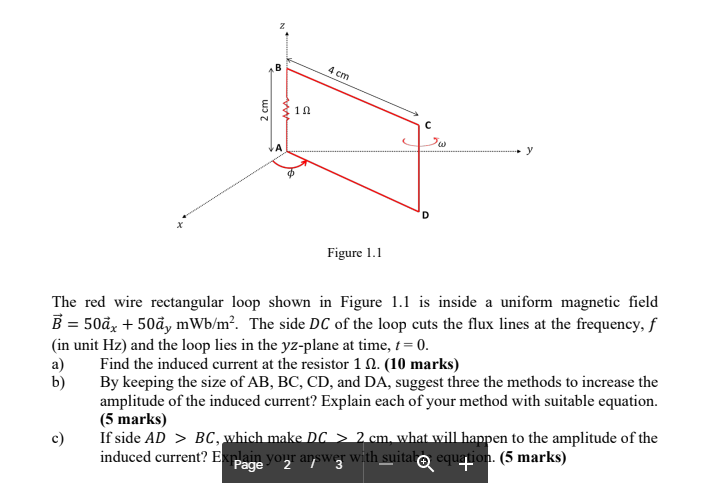This Homework Help Question: "electromagnetic" No answers yet.

We need 9 more requests to produce the answer to this homework help question. Share with your friends to get the answer faster!

1 /10 have requested the answer to this homework help question.

Once 10 people have made a request, the answer to this question will be available in 1-2 days.
All students who have requested the answer will be notified once they are available.

#### Earn Coin

Coins can be redeemed for fabulous gifts.

Similar Homework Help Questions
• ### 8. (30) A plane electromagnetic direction. The electric polarization is parallel to i. The amplitude of...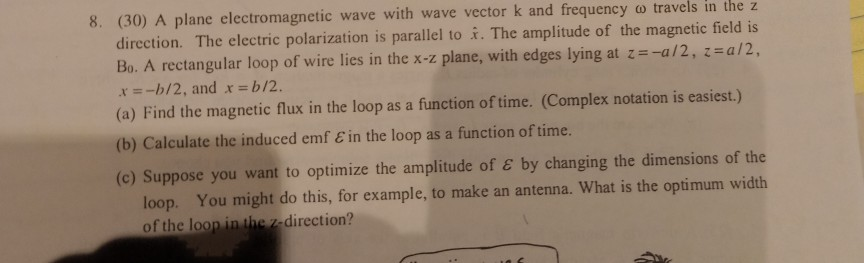8. (30) A plane electromagnetic direction. The electric polarization is parallel to i. The amplitude of the magnetic field is Bo. A rectangular loop of wire lies in the x-z plane, with edges lying at z-a/2, z=al2, -b12, and x -b/2. (a) Find the magnetic flux in the loop wave with wave vectork and frequency o travels in the z as a function of time. (Complex notation is easiest.) (b) Calculate the induced emf E in the loop as a...

• ### I. A rectangular loop rotates about the z axis at an angular speed of ω-5 rads...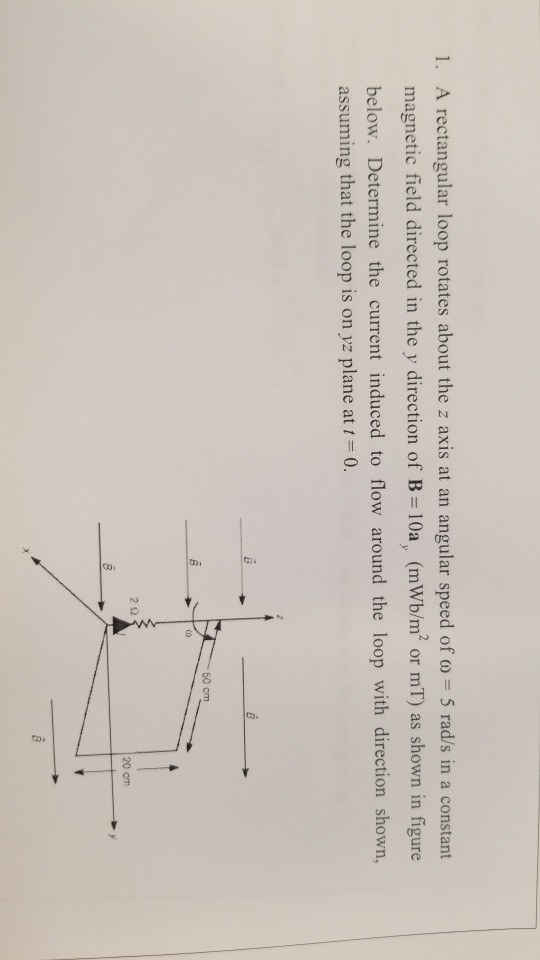I. A rectangular loop rotates about the z axis at an angular speed of ω-5 rads in a constant magnetic field directed in the y direction of B = 10a) (mwb/m2 or mT) as shown in figure below. Determine the current induced to flow around the loop with direction shown, assuming that the loop is on yz plane at 0. 50 cm 2Ω 20 cm

• ### Al. A 1 kVA single-phase step-down transformer is connected to a 220 V, 50 Hz A.C....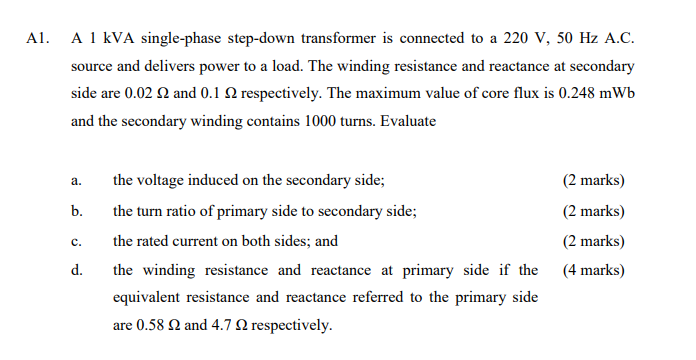Al. A 1 kVA single-phase step-down transformer is connected to a 220 V, 50 Hz A.C. source and delivers power to a load. The winding resistance and reactance at secondary side are 0.02 2 and 0.1 12 respectively. The maximum value of core flux is 0.248 mWb and the secondary winding contains 1000 turns. Evaluate a. b. the voltage induced on the secondary side; the turn ratio of primary side to secondary side; the rated current on both sides; and...

• ### The rotating loop in an AC generator is a square 13.0 cm on a side. It...

The rotating loop in an AC generator is a square 13.0 cm on a side. It is rotated at 75.0 Hz in a uniform field of 0.800 T. Calculate the following quantities as functions of time t, where t is in seconds. (a) the flux through the loop mT·m2 (b) the emf induced in the loop V (c) the current induced in the loop for a loop resistance of 2.00 Ω A (d) the power delivered to the loop W...

• ### The rotating loop in an AC generator is a square 10.0 cm on a side. It...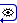The rotating loop in an AC generator is a square 10.0 cm on a side. It is rotated at 55.0 Hz in a uniform field of 0.800 T. Calculate the following quantities as functions of time t, where t is in seconds. (a) the flux through the loop mT·m2 (b) the emf induced in the loop V (c) the current induced in the loop for a loop resistance of 2.00 Ω A (d) the power delivered to the loop W...

• ### In a region with a constant magnetic field as shown, there are two conducting frictionless horizontal...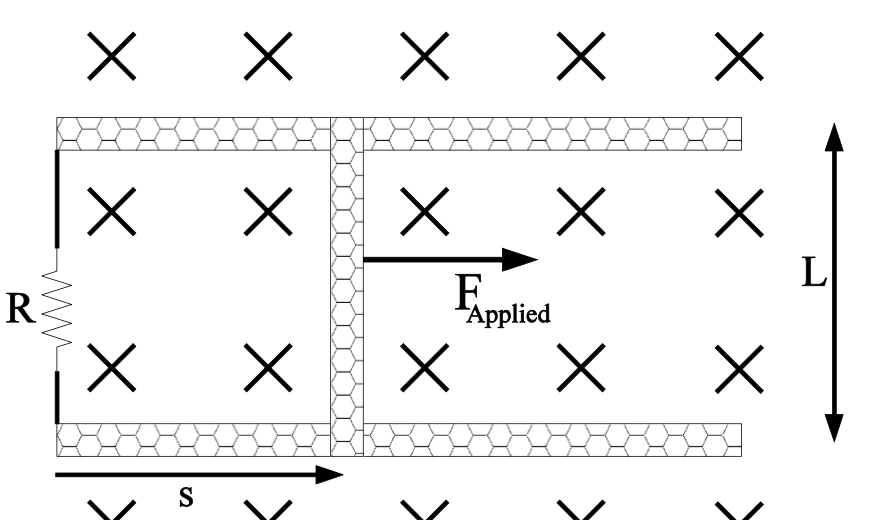In a region with a constant magnetic field as shown, there are two conducting frictionless horizontal rails, a resistor and some conducting wire along the left side and a conducting bar on the right side that is free to slide left or right. The bar is initially at rest but at time zero a force is applied to the bar pulling it to the right so that the distance s increases with time. a. Magnetic flux through the loop increases...

• ### This question is related to real transformers. Kindly show all working. Assume at full load. 25KVA...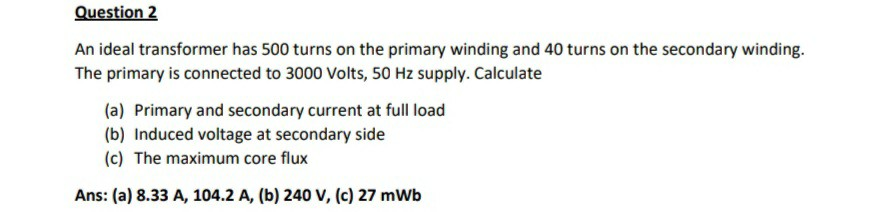This question is related to real transformers. Kindly show all working. Assume at full load. 25KVA Question 2 An ideal transformer has 500 turns on the primary winding and 40 turns on the secondary winding. The primary is connected to 3000 Volts, 50 Hz supply. Calculate (a) Primary and secondary current at full load (b) Induced voltage at secondary side (c) The maximum core flux Ans: (a) 8.33 A, 104.2 A, (b) 240 V, (c) 27 mWb

• ### can you calculate and explain why? thanks (a) A triangular loop with an area of 4 m2, positioned in the x-y plane, i...can you calculate and explain why? thanks (a) A triangular loop with an area of 4 m2, positioned in the x-y plane, is situated in air in a time-varying magnetic field, as shown in Figure 2. The loop includes a resistor of 10 Ω. The magnetic flux density B is given as t+1 6) Determine the induced emf (electromotive force) in the triangular loop. (Gi) Determine the induced current in the triangular loop, assuming that the wire resistance is negligible....

• ### problem #4 #7 4. Problem A long wire is bent to form a rectangular section and is placed in a region with a uniform...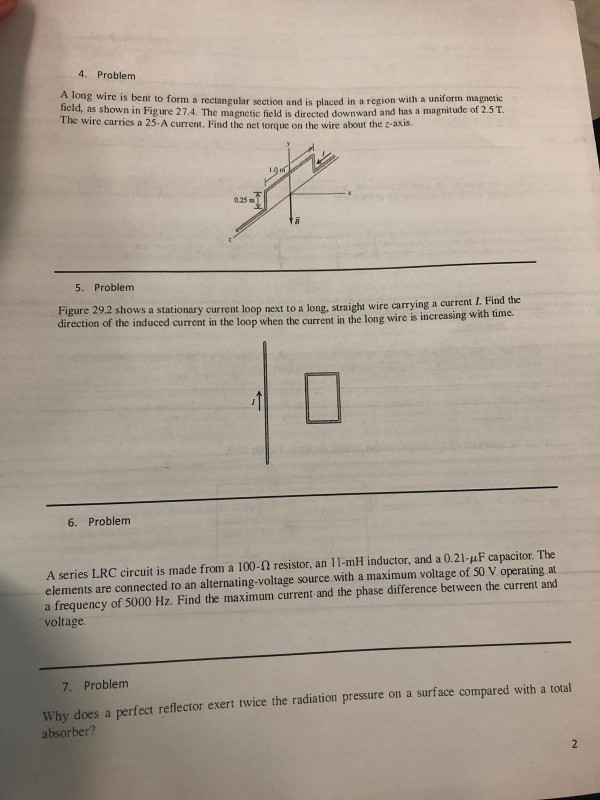problem #4 #7 4. Problem A long wire is bent to form a rectangular section and is placed in a region with a uniform magnetic id, as shown in Fig ure 27.4. The magnetic field is directed downward and has a magnitude of 2.5T. The wire carries a 25- A current. Find the net torque on the wire about the z-axis. 025m 5. Problem Figure 29.2 shows a stationary current loop next to a long, straight wire carrying a current...

• ### A rectangular loop of wire has a resistor with a resistance R=50.00 Ω on the left vertical wire, ...

A rectangular loop of wire has a resistor with a resistance R=50.00 Ω on the left vertical wire, while the light vertical wire can be. over back and forth to make the loop larger or smaller. The vertical wires (the light of the rectangle) are 0.200 cm in length, and the entire circuit is in an area of space where a magnetic field of magnitude 0.750T and pointing out of the page is present. a) Draw the situation, including the...

Free Homework Help App